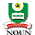# HOW TO CALCULATE NOUN E-EXAMINATION SCORE AND TMA

Exam is around the corner and majority of candidates wants to know how NOUN grading system is when it comes to calculating Exam Score and TMA.

Follow me as I teach you….

NOUN Exam is over 70

NOUN TMA is over 30
TOTAL: 100

TMA means Tutor Marked Assignment. For your registered, you are entitled to 4TMA, of which you must submit before end of the semester exam. After submitting, it will be graded over 10, but the best three TMA will be considered and be chosen, which makes it 30.

Take for instance: Let’s take GST102 as a course case study. Let’s say, TMA 1 – 9.50
TMA 2 – 10.0
TMA 3 – 9.00
TMA 4 – 10.0

The best 3 are 9.50, 10.0 and 10,0 – which is equal to 29.50 over 30

Now the question is, how do i calculate my exam score plus my TMA score?

I must tell you, it’s very simple!

You should note that, all NOUN questions doesn’t Have the same number of questions, some have 100, 120, 150 and 35. The courses with 35 are most of the time MTH courses. 100 and 120 questions are 2unit courses
150 questions are 3 unit courses

For Example: (GST102)
After GST102 exam, you checked your score and you scored 95 out of 120 questions. And in your TMA for GST102, like I said earlier, you scored 29.50/30.

To calculate your total score over 100, use this formula:

Formula:
x/y x 70/1
That is, let x = what you score in the exam, and y = total number of exam question, which simple means, EXAM SCORE divided by NUMBER OF EXAM QUESTION multiplied by 70.

Exam score (x) = 95

Total number of exam question (y) = 120

Therefore, it will be 95/120 x 70

The result is 55.41 (NB: your TMA score is 29.50).

Now, add 55.41 and 29.50 – Result is 84.91

Which means, GST102 = A

also, note, you’ll follow th same way to calculate if your exam question is 100 ot 150.

But in the case of 35 questions, all you need to do is multiply whatever you score by 2.

That is, if you score 20/35, it will be 20 x 2, which is equal to 40, meaning that you score 40/70. But sometimes, it will have been programmed in such a way the calculation would have been done. You’ll know if it is.

You’ll see your score over 70.

0-39 — F
40-44 — E
45-49 — D
50-59 — C
60-69 — B
70-100 – A

##### 5 on: "HOW TO CALCULATE NOUN E-EXAMINATION SCORE AND TMA"
1.1.Also you can calculate your scores automatically with this calculator, try it.

2.What about 85/120 and 73.33/100 can you help me calculate it?

3.4.